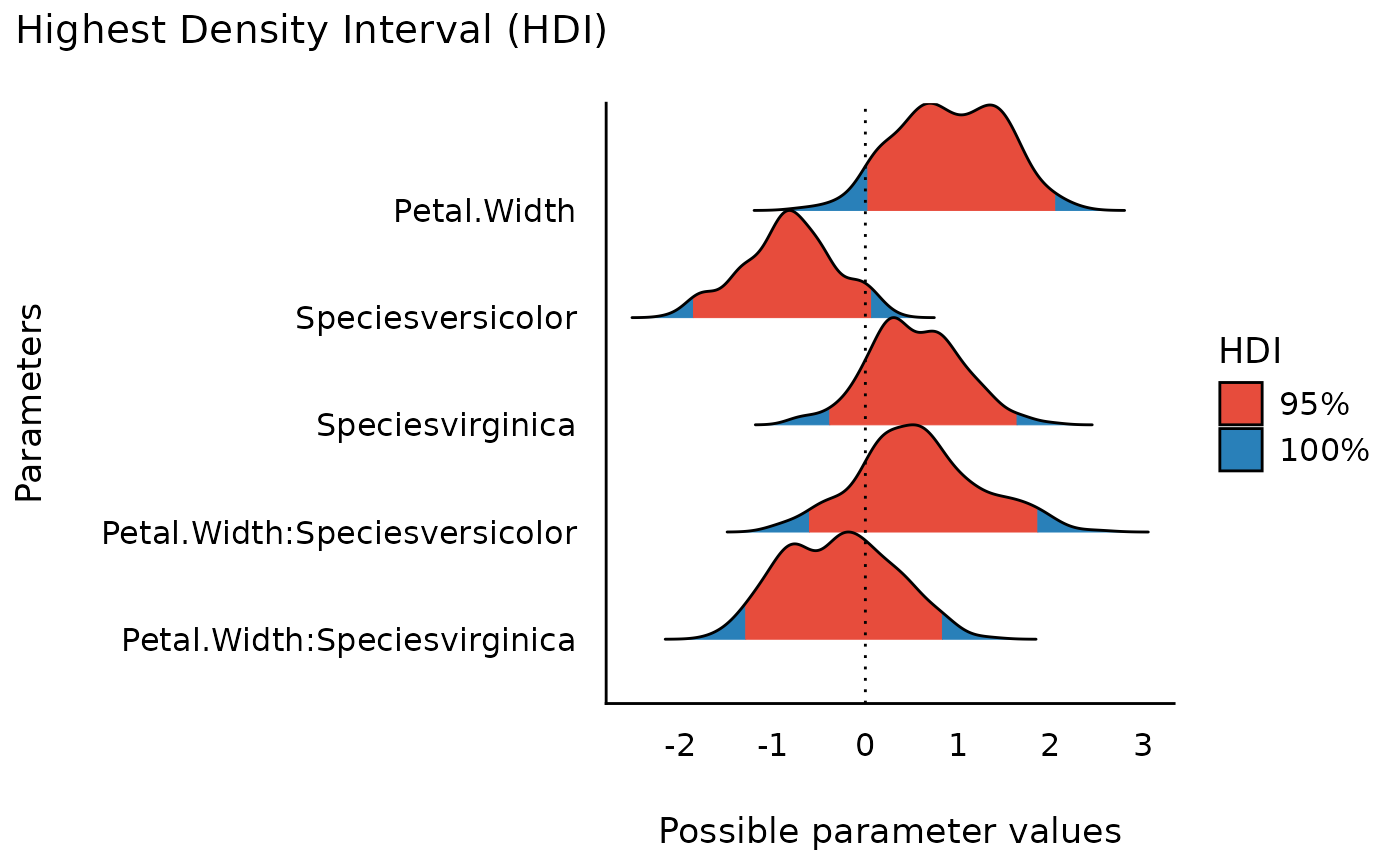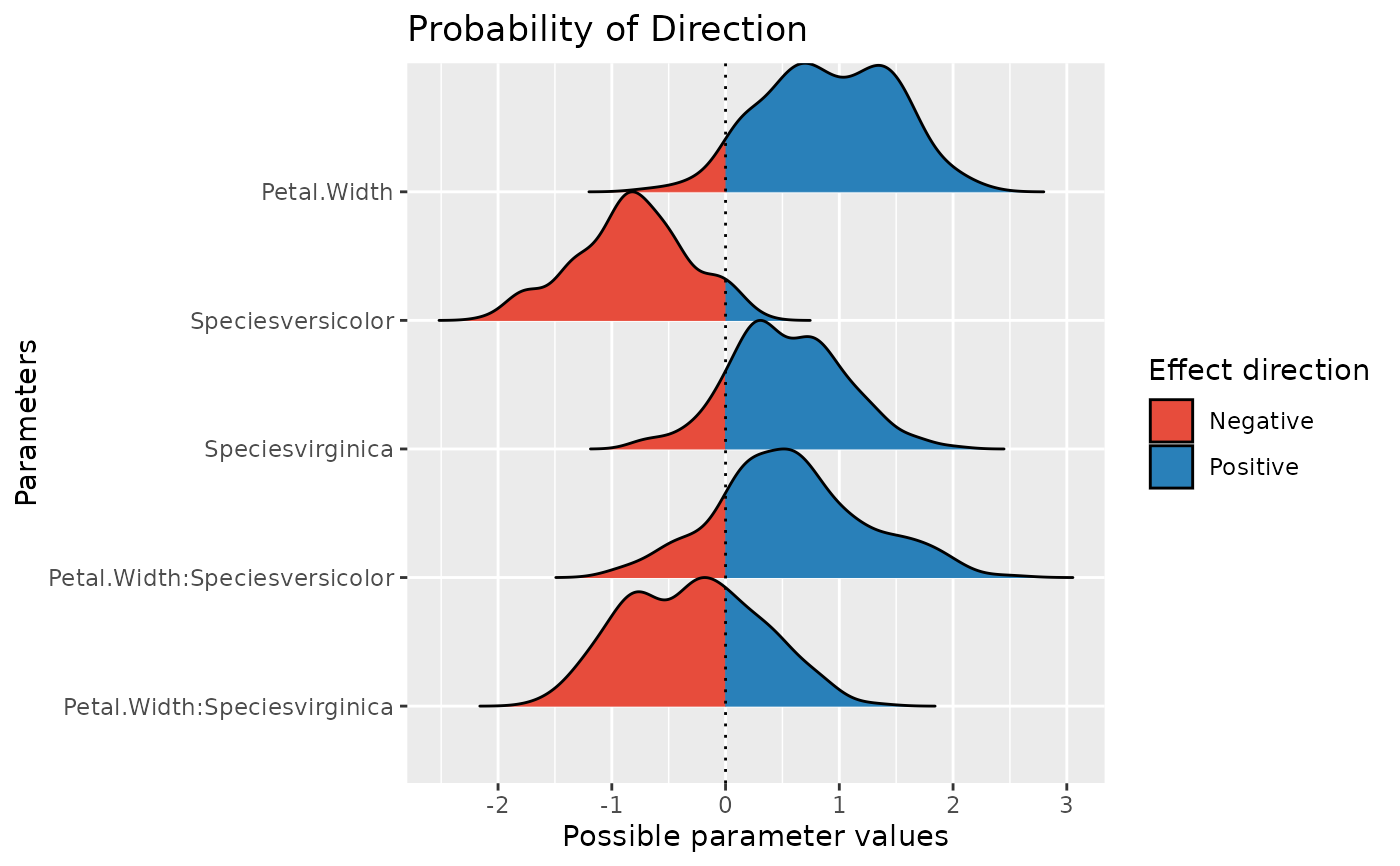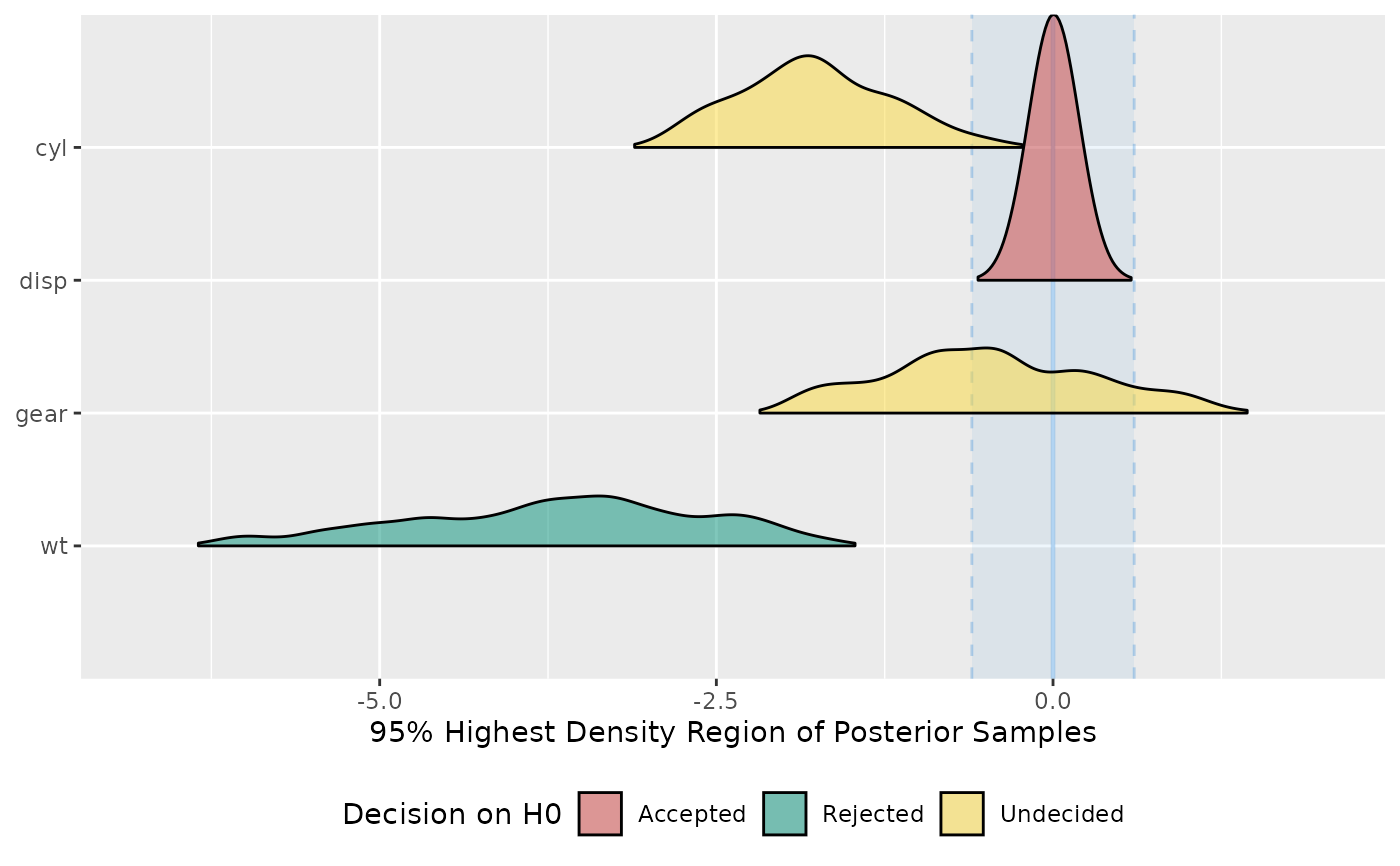data_plot() extracts and transforms an object for plotting, while plot() visualizes results of functions from different packages in easystats-project. See the documentation for your object's class:

## Usage

data_plot(x, data = NULL, ...)

## Arguments

x

An object.

data

The original data used to create this object. Can be a statistical model.

...

Arguments passed to or from other methods.

## Details

data_plot() is in most situation not needed when the purpose is plotting, since most plot()-functions in see internally call data_plot() to prepare the data for plotting.

Many plot()-functions have a data-argument that is needed when the data or model for plotting can't be retrieved via data_plot(). In such cases, plot() gives an error and asks for providing data or models.

Most plot()-functions work out-of-the-box, i.e. you don't need to do much more than calling plot(<object>) (see 'Examples'). Some plot-functions allow to specify arguments to modify the transparency or color of geoms, these are shown in the 'Usage' section.

## Examples

library(bayestestR)
library(rstanarm)

model <<- suppressWarnings(stan_glm(
Sepal.Length ~ Petal.Width * Species,
data = iris,
chains = 2, iter = 200, refresh = 0
))

x <- rope(model, verbose = FALSE)
plot(x)x <- hdi(model)
plot(x) + theme_modern()data <- rnorm(1000, 1)
x <- p_direction(data)
plot(x)x <- p_direction(model, verbose = FALSE)
plot(x)model <<- suppressWarnings(stan_glm(
mpg ~ wt + gear + cyl + disp,
chains = 2,
iter = 200,
refresh = 0,
data = mtcars
))
x <- equivalence_test(model, verbose = FALSE)
plot(x)
#> Picking joint bandwidth of 0.168